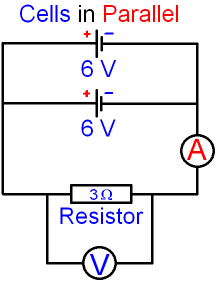gcsescience.com                                       26                                       gcsescience.com

Electricity

Parallel Circuits

What happens to the Voltage and Current
in a Circuit when Cells are connected in Parallel?

In the circuit below, it has been shown that
the current is 2 amps and the voltage is 6 volts.The circuit above has one cell. What happens
if two cells are connected in parallel?

When an identical cell is placed in parallel with
the original cell, the current stays the same because
the total voltage of the circuit stays the same.In the circuit above with two 6 volt cells in parallel,
the current is still 2 amps and the voltage is still 6 volts.

The two cells in parallel together supply electricity
for twice as long as one cell before they both run out.

Compare this with cells in series.

gcsescience.com      Physics Quiz      Index      Electricity Quiz      gcsescience.com